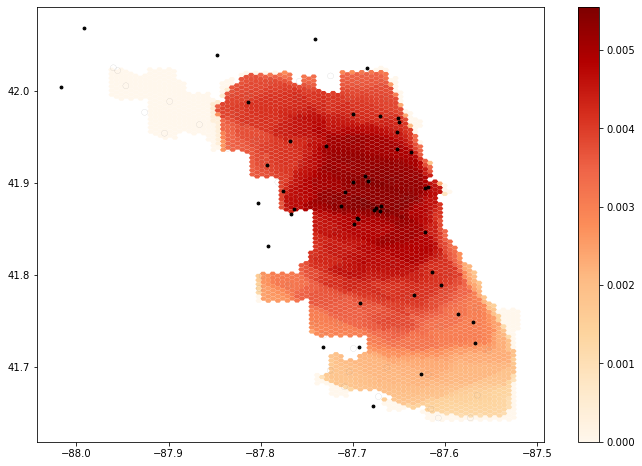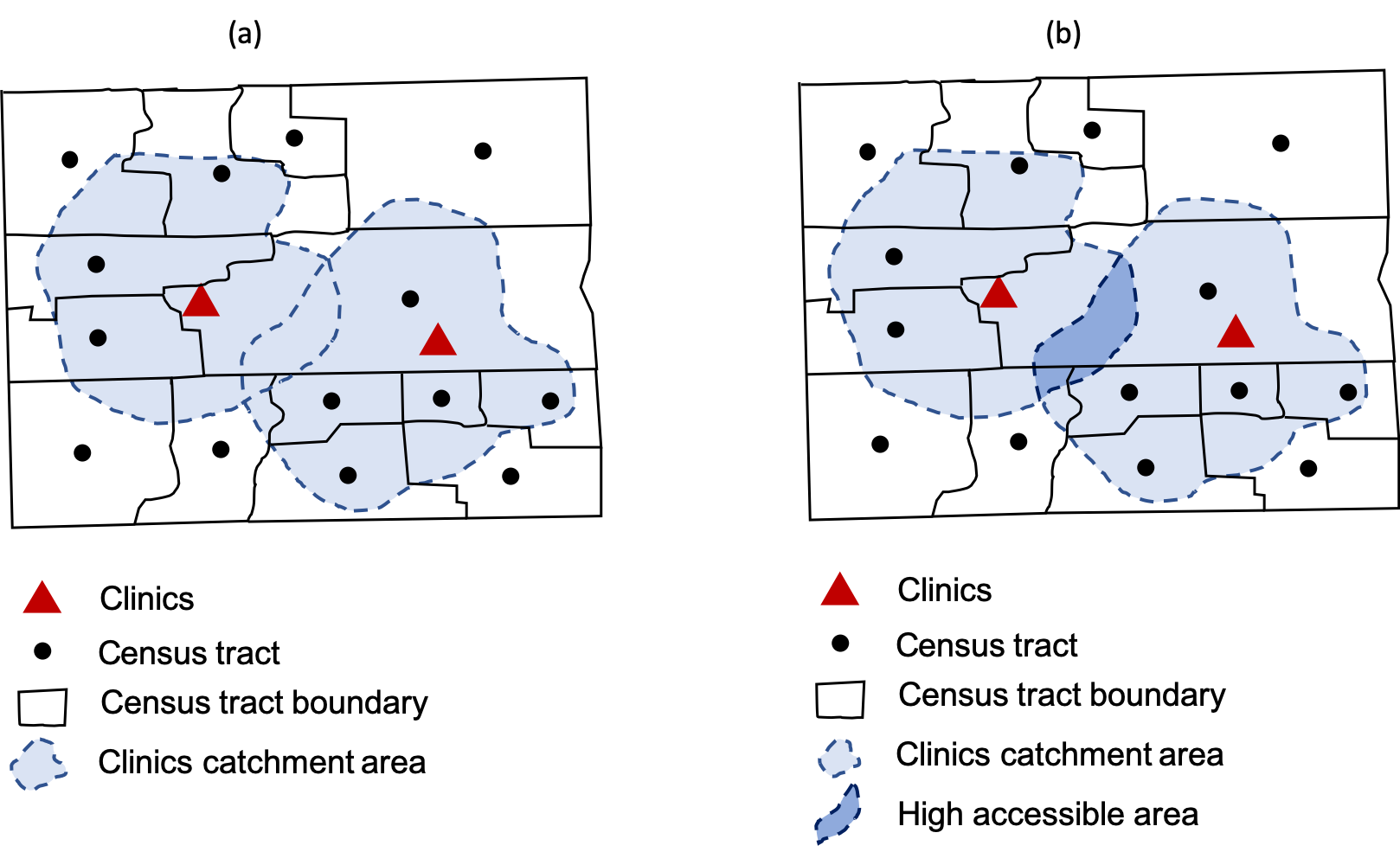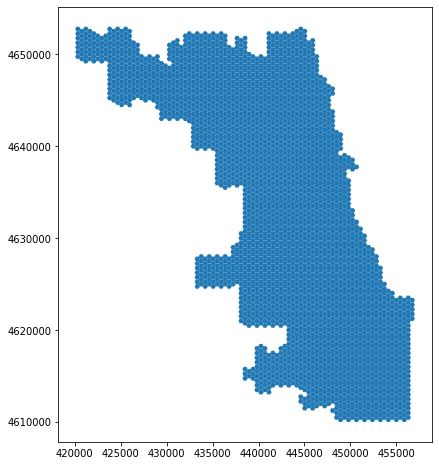Rapidly Measuring Spatial Accessibility of COVID-19 Healthcare Resources: A Case Study of Illinois, USA

**Author**: Jeon-Young Kang 1,2, Alexander Michels 1,3, Fanzheng Lyu1,2, Shaohua Wang1,2 , Nelson Agbodo4, Vincent Freeman5, Shaowen Wang1,2,3,*

1 CyberGIS Center for Advanced Digital and Spatial Studies, University of Illinois at UrbanaChampaign, Urbana, Illinois
2 Department of Geography and Geographic Information Science, University of Illinois at Urbana-Champaign, Urbana, Illinois
3 Illinois Informatics Institute, University of Illinois at Urbana-Champaign, Urbana, Illinois
4 Division of Health Data and Policy, Illinois Department of Public Health, Springfield, Illinois
5 Division of Epidemiology and Biostatistics, School of Public Health, University of Illinois at Chicago, Chicago, Illinois

* Corresponding author: shaowen@illinois.edu### Reference¶

Kang, J. Y., Michels, A. C., Lyu, F., Wang, S., Agbodo, N., Freeman, V. L., & Wang, S. (2020). Rapidly Measuring Spatial Accessibility of COVID-19 Healthcare Resources: A Case Study of Illinois, USA. medRxiv https://doi.org/10.1101/2020.05.06.20093534

### Objectives¶

This aims to measure spatial access for people to hospitals in Illinois. The spatial accessibiilty is measured by the use of an enhanced two-step floating catchment area (E2FCA) method (Luo & Qi, 2009), which is an outcome of interactions between demands (i.e, # of potential patients; people) and supply (i.e., # of beds or physicians). As a result, the map of spatial accessibility will be produced. It identifies which regions need more healthcare resources, such as the number of beds or physicians. This notebook would serve as a guideline of which regions need more beds for fighting against COVID-19.

### Data¶

To perform the ESFCA method, three types of data are required, as follows: (1) road network, (2) population, and (3) hospital information. The road network can be obtained from the OpenStreetMap Python Library, called OSMNX. The population data is available on the American Community Survey. Lastly, hosptial information is also publically available on the Homelanad Infrastructure Foundation-Level Data.

### Method - An enhanced two-step floating catchment area (E2FCA)¶

The catchement area for each hospital will be delineated by travel times. People living in the overlappying regions by multiple hospitals' catchment area are more accessible to hospitals than people living in other places.### Codes¶

import necessary librareis to run this model.

In :
import pandas as pd
import numpy as np
import geopandas as gpd
import networkx as nx
import osmnx as ox
from shapely.geometry import Point, LineString, Polygon
import matplotlib.pyplot as plt
from tqdm import tqdm
import multiprocessing as mp
import itertools, os, time, warnings

warnings.filterwarnings("ignore")


The followings are necessary functions to run the E2FCA method.

In :
# network setting
# the road networks obtained open street map often do not include speed limits at some road segments.
# So, this function helps to set up the speed limits on each road segment
def network_setting(network):
for u, v, k, data in tqdm(G.edges(data=True, keys=True),position=0):
if 'maxspeed' in data.keys():
speed_type = type(data['maxspeed'])
if (speed_type==str):
if len(data['maxspeed'].split(','))==2:
data['maxspeed']=float(data['maxspeed'].split(','))
elif data['maxspeed']=='signals':
data['maxspeed']=35.0 # drive speed setting as 35 miles
else:
data['maxspeed']=float(data['maxspeed'].split())
else:
data['maxspeed']=float(data['maxspeed'].split())

else:
data['maxspeed']= 35.0 #miles

data['maxspeed_meters'] = data['maxspeed']*26.8223 # convert mile to meter
data['time'] = float(data['length'])/ data['maxspeed_meters']

print('network setting is done')
return(network)

In :
# set hospitals on OSMNX street network
def hospital_setting (hospitals):
hospitals['nearest_osm']=None
hospitals['value'] = None
for i in tqdm(hospitals.index, desc="Find the nearest osm from hospitals", position=0):
point = [hospitals['LATITUDE'][i], hospitals['LONGITUDE'][i]]
nearest_osm = ox.get_nearest_node(G, point, method='euclidean') # find the nearest node from hospital location
hospitals['nearest_osm'][i] = nearest_osm
hospitals['value'][i] = hospitals['BEDS'][i]
print ('hospital setting is done')

return(hospitals)

In :
# to estimate the centroids of zipcode
def pop_centroid (pop_data, pop_type):
pop_data = pop_data.to_crs({'init': 'epsg:4326'})

if pop_type== "pop":
pop_data=pop_data[pop_data['pop']>=0]

if pop_type== "covid":
pop_data=pop_data[pop_data['cases']>=0]

pop_cent = pop_data.centroid # it make the polygon to the point without any other information

pop_centroid = gpd.GeoDataFrame()
i = 0
for point in tqdm(pop_cent, desc='Pop Centroid File Setting', position=0):
zip_code = pop_data.iloc[i].ZCTA5CE10

if pop_type== "pop":
pop = pop_data.iloc[i]['pop']

if pop_type=="covid":
pop = pop_data.iloc[i]['cases']

geometry = point
pop_centroid = pop_centroid.append({'zip_code': zip_code,
'pop': pop,
'geometry': geometry}, ignore_index=True)
i = i+1

print ('population setting is done')
return(pop_centroid)

In :
def hospital_measure_acc(_thread_id, hospital, pop_data, network):
## find nodes within 10 mins
node_points_10 = [Point((data['x'], data['y'])) for node, data in road_network_10.nodes(data=True)]
polygon_10 = gpd.GeoSeries(node_points_10).unary_union.convex_hull ## to create convex hull
poly_10 = gpd.GeoDataFrame(gpd.GeoSeries(polygon_10)) ## change polygon to geopandas
poly_10 = poly_10.rename(columns={0:'geometry'}).set_geometry('geometry')

## find nodes within 20 mins
node_points_20 = [Point((data['x'],data['y'])) for node, data in road_network_20.nodes(data=True)]
polygon_20 = gpd.GeoSeries(node_points_20).unary_union.convex_hull ## to create convex hull
poly_20 = gpd.GeoDataFrame(gpd.GeoSeries(polygon_20)) ## change polygon to geopandas
poly_20 = poly_20.rename(columns={0:'geometry'}).set_geometry('geometry')

## find nodes within 30 mins
node_points_30 = [Point((data['x'],data['y'])) for node, data in road_network_30.nodes(data=True)]
polygon_30 = gpd.GeoSeries(node_points_30).unary_union.convex_hull ## to create convex hull
poly_30 = gpd.GeoDataFrame(gpd.GeoSeries(polygon_30)) ## change polygon to geopandas
poly_30 = poly_30.rename(columns={0:'geometry'}).set_geometry('geometry')

#extract not overlapping regions between 10mins and 20 mins - > 10-20 mins convex hull
poly_10_20 = gpd.overlay(poly_20, poly_10, how='difference')
#extract not overlapping regions between 20mins and 30 mins; 20-30 mins convex hull
poly_20_30 = gpd.overlay(poly_30, poly_20, how='difference')

num_pops = []

for j in pop_data.index:
point = pop_data['geometry'][j]

if (point.within(polygon_10)): # find the centroid within 10 mins convex hull
pop_value = pop_data['pop'][j]
num_pops.append(pop_value)

if len(poly_10_20)>0: # to exclude the weirdo (convex hull is not polygon)
if (point.within(poly_10_20.iloc['geometry'])): # find the centroid within 10- 20 mins convex hull
pop_value = round(pop_data['pop'][j]*0.68)
num_pops.append(pop_value)

if len(poly_20_30)>0: # to exclude the weirdo (convex hull is not polygon)
if (point.within(poly_20_30.iloc['geometry'])): # find the centroid within 20-30 mins convex hull
pop_value = round(pop_data['pop'][j]*0.22)
num_pops.append(pop_value)

total_pop = sum(num_pops)

poly_10['time']=10
poly_10['total_pop'] = total_pop
poly_10['hospital_value']=hospital['value'] # number of beds
poly_10['value'] = poly_10['hospital_value']/poly_10['total_pop'] # proportion of # of beds over pops in 10 mins

poly_10_20['time']=20
poly_10_20['total_pop']= total_pop
poly_10_20['hospital_value']=hospital['value'] # number of beds
poly_10_20['value'] = poly_10_20['hospital_value']/poly_10_20['total_pop'] # proportion of # of beds over pops in 10-20 mins

poly_20_30['time']=30
poly_20_30['total_pop']= total_pop
poly_20_30['hospital_value']=hospital['value'] # number of beds
poly_20_30['value'] = poly_20_30['hospital_value']/poly_20_30['total_pop'] # proportion of # of beds over pops in 20-30 mins

# set up the projection (WGS 84)
poly_10.crs ={'init': 'epsg:4326'}
poly_10_20.crs ={'init': 'epsg:4326'}
poly_20_30.crs ={'init': 'epsg:4326'}

# change the projection to UTM (for matching with GRID FILES)
poly_10 = poly_10.to_crs({'init':'epsg:32616'})
poly_10_20 = poly_10_20.to_crs({'init':'epsg:32616'})
poly_20_30 = poly_20_30.to_crs({'init':'epsg:32616'})

def hospital_acc_unpacker(args):
return hospital_measure_acc(*args)

def measure_acc_par (hospitals, pop_data, network, num_proc = 4):
##distance weight = 1, 0.68, 0.22

output_10 = gpd.GeoDataFrame()
output_20 = gpd.GeoDataFrame()
output_30 = gpd.GeoDataFrame()

pool = mp.Pool(processes = num_proc)
hospital_list = [ hospitals.iloc[i] for i in range(len(hospitals)) ]
results = pool.map(hospital_acc_unpacker, zip(range(len(hospital_list)), hospital_list, itertools.repeat(pop_data), itertools.repeat(network)))
results.sort()
results = [ r for r in results ]

for i in range(len(results)):
output_10 = output_10.append(results[i], sort=False)
output_20 = output_20.append(results[i], sort=False)
output_30 = output_30.append(results[i], sort=False)

output_10 = output_10[output_10['value']!=float('inf')] #some hospitals do not have centroid within its catchment area.
output_20 = output_20[output_20['value']!=float('inf')]
output_30 = output_30[output_30['value']!=float('inf')]
return output_10, output_20, output_30

In :
from collections import Counter
def overlap_calc(_id, poly, grid_file, weight):
if type(poly.iloc['value'])!=type(None):
value = float(poly['value'])*weight
intersect = gpd.overlay(grid_file, poly, how='intersection')
intersect['overlapped']= intersect.area
intersect['percent'] = intersect['overlapped']/intersect['area']
intersect=intersect[intersect['percent']>=0.5]
intersect_region = intersect['id']
value_dict = Counter()
for intersect_id in intersect_region:
try:
value_dict[intersect_id] +=value
except:
value_dict[intersect_id] = value
return(_id, value_dict)

def overlap_calc_unpacker(args):
return overlap_calc(*args)

def overlapping_function (grid_file, acc10, acc20, acc30, num_proc = 4):

grid_file['value']=0
weights = [1.0, 0.68, 0.22]
time_stamps = [ time.perf_counter() ]

pool = mp.Pool(processes = num_proc)
acc_list = [ acc10[i:i+1] for i in range(len(acc10)) ] + [ acc20[i:i+1] for i in range(len(acc20)) ] + [ acc30[i:i+1] for i in range(len(acc30)) ]
acc_weights = [weights]*len(acc10) + [weights]*len(acc20) + [weights]*len(acc30)
results = pool.map(overlap_calc_unpacker, zip(range(len(acc_list)), acc_list, itertools.repeat(grid_file), acc_weights))
results.sort()
results = [ r for r in results ]
res = results
for result in results[1:]:
res += result
for intersect_id, value in res.items():
grid_file.loc[grid_file['id']==intersect_id, 'value'] += value
time_stamps.append(time.perf_counter())
print(f"ACC Ran in: {(time_stamps[len(time_stamps)-1]-time_stamps[len(time_stamps)-2]):0.4f} seconds")

grid_file.to_crs({'init': 'epsg:4326'})
return(grid_file)

In :
def output_map (output_grid, base_map, hospitals):
ax=output_grid.plot(column='normal_value', cmap='Blues', figsize=(12,8), legend=True, zorder=1)
base_map.plot(ax=ax, facecolor="none", edgecolor='black', lw=0.2)
ax.text(-88, 41.68, "Legend", fontsize=13)
ax.text(-88, 41.65, "+ : hospital", fontsize=12)
if hospitals is not None:
ax.scatter(hospitals.LONGITUDE, hospitals.LATITUDE, marker="+",zorder=1, c='black', s=50)


### Population and COVID-19 Cases Data by County¶

In :
pop_data = gpd.read_file('./Data/PopData/Chicago_ZIPCODE.shp')

Out:
ZCTA5CE10 County State Join ZONE ZONENAME FIPS pop cases geometry
0 60660 Cook County IL Cook County IL IL_E Illinois East 1201 43242 78 POLYGON ((-87.65049 41.99735, -87.65029 41.996...
1 60640 Cook County IL Cook County IL IL_E Illinois East 1201 69715 117 POLYGON ((-87.64645 41.97965, -87.64565 41.978...
2 60614 Cook County IL Cook County IL IL_E Illinois East 1201 71308 134 MULTIPOLYGON (((-87.67703 41.91845, -87.67705 ...
3 60712 Cook County IL Cook County IL IL_E Illinois East 1201 12539 42 MULTIPOLYGON (((-87.76181 42.00465, -87.76156 ...
4 60076 Cook County IL Cook County IL IL_E Illinois East 1201 31867 114 MULTIPOLYGON (((-87.74782 42.01540, -87.74526 ...

### Hospital Data¶

Note that 999 is treated as a "NULL"/"NA" so these hospitals are filtered out. We also only kept hospitals which would treat civilian COVID-19 cases, meaning we filtered out psychiatric, military, and rehab sites.

In :
hospitals = gpd.read_file('./Data/HealthCareData/Hospital_Chicago.shp')
hospitals = hospitals[hospitals['BEDS']!=-999]
hospitals = hospitals[hospitals["TYPE"].isin(["GENERAL ACUTE CARE", "CRITICAL ACCESS","LONG TERM CARE"])]

Out:
OBJECTID ID NAME ADDRESS CITY STATE ZIP ZIP4 TELEPHONE TYPE ... WEBSITE STATE_ID ALT_NAME ST_FIPS OWNER TTL_STAFF BEDS TRAUMA HELIPAD geometry
2 1516 0003660628 ROSELAND COMMUNITY HOSPITAL 45 W 111TH STREET CHICAGO IL 60628 NOT AVAILABLE (773) 995-3000 GENERAL ACUTE CARE ... http://www.roselandhospital.org 140068 NOT AVAILABLE 17 NON-PROFIT -999 115 NOT AVAILABLE N POINT (-87.62536 41.69223)
3 1517 0002360302 WEST SUBURBAN MEDICAL CENTER 3 ERIE COURT OAK PARK IL 60302 NOT AVAILABLE (708) 383-6200 GENERAL ACUTE CARE ... http://www.westsuburbanmc.com/Home.aspx 140049 NOT AVAILABLE 17 PROPRIETARY -999 172 NOT AVAILABLE N POINT (-87.77618 41.89140)
4 1890 0002860402 MACNEAL HOSPITAL 3249 SOUTH OAK PARK AVENUE BERWYN IL 60402 NOT AVAILABLE (708) 783-9100 GENERAL ACUTE CARE ... http://www.macneal.com 140054 NOT AVAILABLE 17 PROPRIETARY -999 371 LEVEL II N POINT (-87.79216 41.83198)
5 1891 0011760634 COMMUNITY FIRST MEDICAL CENTER 5645 W ADDISON CHICAGO IL 60634 NOT AVAILABLE (773) 282-7000 GENERAL ACUTE CARE ... http://www.presencehealth.org 140251 NOT AVAILABLE 17 NON-PROFIT -999 279 NOT AVAILABLE N POINT (-87.76784 41.94545)
6 4116 0007360612 UNIVERSITY OF ILLINOIS HOSPITAL 1740 WEST TAYLOR ST, SUITE 1400 CHICAGO IL 60612 NOT AVAILABLE (312) 996-3900 GENERAL ACUTE CARE ... http://www.hospital.uillinois.edu/ 140150 NOT AVAILABLE 17 GOVERNMENT - STATE -999 483 NOT AVAILABLE N POINT (-87.67052 41.86967)

5 rows × 33 columns

### Generate and Plot Map of Hospitals¶

In :
import folium, random
sites_cat = hospitals['TYPE'].unique().tolist() # Obtain the type of schools
sites_dict = {}
rd_color =["#"+''.join([random.choice('0123456789ABCDEF') for j in range(6)])
for i in range(len(sites_cat))]   # generate random color based on the types of schools
for i in range(len(sites_cat)):
hospitals.loc[hospitals['TYPE'] == sites_cat[i], 'color'] = rd_color[i]   # set value for color column
sites_dict[sites_cat[i]] = rd_color[i]
m = folium.Map(location=[41.85, -87.65], tiles='cartodbpositron', zoom_start=11)
for i in range(0, len(hospitals)):
folium.CircleMarker(
location=[hospitals.iloc[i]['geometry'].y, hospitals.iloc[i]['geometry'].x],
popup="{}\n{}".format(hospitals.iloc[i]['NAME'],hospitals.iloc[i]['BEDS']),
color='grey',
weight=0.1,
fill=True,
fill_color=hospitals.iloc[i]['color'],
fill_opacity=0.6,
legend_name = 'Hospitals'

legend_html =   '''<div style="position: fixed; width: 20%; heigh: auto;
bottom: 10px; left: 10px;
solid grey; z-index:9999; font-size:14px;
">&nbsp; Legend<br>'''
color_leg = ""
for key, value in sites_dict.items():
color_leg += '&nbsp; <i class="fa fa-circle" style="font-size:10px;color: '+ value + '"></i>' +'&nbsp;'
color_leg += key + '<br>'
legend_html += color_leg + '</div>'''
m.save('img/Chicago_hospitals_interactive_map.html')


### Load and Plot Hexagon Grids (500-meter resolution)¶

In :
grid_file = gpd.read_file('./Data/GridFile/Chicago_Grid.shp')
grid_file.plot(figsize=(8,8))

Out:
<matplotlib.axes._subplots.AxesSubplot at 0x1a22483710>### Load and Plot the Street Network¶

In :
if not os.path.exists("Data/Chicago_Network.graphml"):
G = ox.graph_from_place('Chicago', network_type='drive')
ox.save_graphml(G, 'Chicago_Network.graphml', folder="Data")
else:

100%|██████████| 75957/75957 [00:00<00:00, 622165.62it/s]

network setting is done